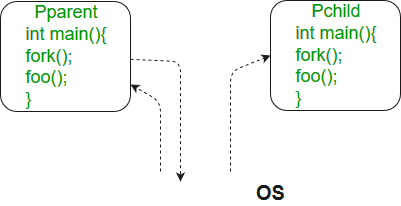GeeksforGeeks App
Open AppBrowser
Continue

# fork() in C

The Fork system call is used for creating a new process in Linux, and Unix systems, which is called the child process, which runs concurrently with the process that makes the fork() call (parent process). After a new child process is created, both processes will execute the next instruction following the fork() system call.

The child process uses the same pc(program counter), same CPU registers, and same open files which use in the parent process. It takes no parameters and returns an integer value.

Below are different values returned by fork().

• Negative Value: The creation of a child process was unsuccessful.
• Zero: Returned to the newly created child process.
• Positive value: Returned to parent or caller. The value contains the process ID of the newly created child process.Note: fork() is threading based function, to get the correct output run the program on a local system.

Please note that the above programs don’t compile in a Windows environment.

## C

 `#include ``#include ;``#include ;``int` `main()``{` `    ``// make two process which run same``    ``// program after this instruction``    ``fork();` `    ``printf``(``"Hello world!\n"``);``    ``return` `0;``}`

Output

```Hello world!
Hello world!```

## C

 `#include ``#include ``#include ``int` `main()``{``    ``fork();``    ``fork();``    ``fork();``    ``printf``(``"hello\n"``);``    ``return` `0;``}`

Output

```hello
hello
hello
hello
hello
hello
hello
hello```

Explanation

The number of times ‘hello’ is printed is equal to the number of processes created. Total Number of Processes = 2n, where n is the number of fork system calls. So here n = 3, 23 = 8 Let us put some label names for the three lines:

```fork ();   // Line 1
fork ();   // Line 2
fork ();   // Line 3

L1       // There will be 1 child process
/     \     // created by line 1.
L2      L2    // There will be 2 child processes
/  \    /  \   //  created by line 2
L3  L3  L3  L3  // There will be 4 child processes
// created by line 3```

So there is a total of eight processes (new child processes and one original process). If we want to represent the relationship between the processes as a tree hierarchy it would be the following: The main process: P0 Processes created by the 1st fork: P1 Processes created by the 2nd fork: P2, P3 Processes created by the 3rd fork: P4, P5, P6, P7

```             P0
/   |   \
P1    P4   P2
/  \          \
P3    P6         P5
/
P7```

## C

 `#include ``#include ;``#include ;``void` `forkexample()``{``    ``// child process because return value zero``    ``if` `(fork() == 0)``        ``printf``(``"Hello from Child!\n"``);` `    ``// parent process because return value non-zero.``    ``else``        ``printf``(``"Hello from Parent!\n"``);``}``int` `main()``{``    ``forkexample();``    ``return` `0;``}`

Output

```Hello from Child!
Hello from Parent!```

Note: In the above code, a child process is created. fork() returns 0 in the child process and positive integer in the parent process. Here, two outputs are possible because the parent process and child process are running concurrently. So we don’t know whether the OS will first give control to the parent process or the child process.

Parent process and child process are running the same program, but it does not mean they are identical. OS allocates different data and states for these two processes, and the control flow of these processes can be different. See the next example:

## C

 `#include ``#include ``#include ` `void` `forkexample()``{``    ``int` `x = 1;` `    ``if` `(fork() == 0)``        ``printf``(``"Child has x = %d\n"``, ++x);``    ``else``        ``printf``(``"Parent has x = %d\n"``, --x);``}``int` `main()``{``    ``forkexample();``    ``return` `0;``}`

Output

```Parent has x = 0
Child has x = 2```

or

Output

```Child has x = 2
Parent has x = 0```

Here, global variable change in one process does not affect two other processes because the data/state of the two processes is different. And also parent and child run simultaneously so two outputs are possible.

### fork() vs exec()

The fork system call creates a new process. The new process created by fork() is a copy of the current process except for the returned value. On the other hand, the exec() system call replaces the current process with a new program.

## C

 `for` `(i = 0; i < n; i++)``    ``fork();`

The total number of child processes created is (GATE-CS-2008)

(A) n
(B) 2^n – 1
(C) 2^n
(D) 2^(n+1) – 1

See this for a solution.

## C

 `if` `(fork() == 0) {``    ``a = a + 5;``    ``printf``(``"%d, %d\n"``, a, &a);``}``else` `{``    ``a = a –5;``    ``printf``(``"%d, %d\n"``, a, &a);``}`

Let u, v be the values printed by the parent process, and x, y be the values printed by the child process. Which one of the following is TRUE? (GATE-CS-2005)

(A) u = x + 10 and v = y
(B) u = x + 10 and v != y
(C) u + 10 = x and v = y
(D) u + 10 = x and v != y

See this for a solution.

## C

 `#include ``#include ``int` `main()``{``    ``fork();``    ``fork() && fork() || fork();``    ``fork();` `    ``printf``(``"forked\n"``);``    ``return` `0;``}`

See this for the solution

Related Articles :